##### Statistics For Dummies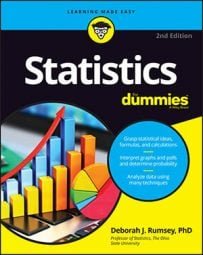You can use a hypothesis test to examine or challenge a statistical claim about a population mean if the variable is numerical (for example, age, income, time, and so on) and only one population or group (such as all U.S. households or all college students) is being studied. For example, a child psychologist says that the average time that working mothers spend talking to their children is 11 minutes per day, on average. The variable — time — is numerical, and the population is all working mothers. Using statistical notation,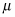represents the average number of minutes per day that all working mothers spend talking to their children, on average.

The null hypothesis is that the population mean,is equal to a certain claimed value,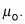The notation for the null hypothesis is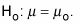So the null hypothesis in this example is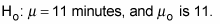The three possibilities for the alternative hypothesis, Ha, are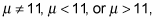depending on what you are trying to show.

If you suspect that the average time working mothers spend talking with their kids is more than 11 minutes, your alternative hypothesis would be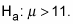To test the claim, you compare the mean you got from your sample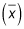with the mean shown in H0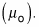To make a proper comparison, you look at the difference between them, and divide by the standard error to take into account the fact that your sample results will vary. This result is your test statistic. In the case of a hypothesis test for the population mean, the test statistic turns out (under certain conditions) to be a z-value (a value from the Z-distribution).

Then you can look up your test statistic on the appropriate table (in this case, you look it up on the below Z-table), and find the probability that results more extreme than those found in your sample could have been found. Typically, if the test statistic Z is positive, you want to find the probability that Z is greater than your test statistic. If you test statistic is negative, you want to find the probability that Z is less than your test statistic.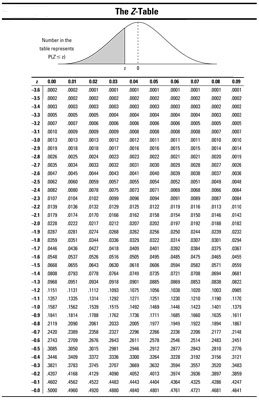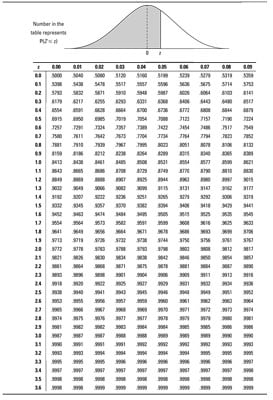The test statistic for testing one population mean (under certain conditions) is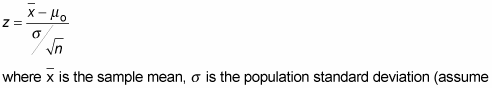for this case that this number is known), and n is the sample size. To calculate the test statistic, do the following:

1. Calculate the sample mean,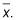2. Find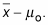3. Calculate the standard error:4. Divide your result from Step 2 by the standard error found in Step 3.

The conditions for using this test statistic are that the population standard deviation,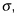is known, and either the population has a normal distribution or the sample size is large enough to use the Central Limit Theorem (n > 30).

For this example, suppose a random sample of 100 working mothers spend an average of 11.5 minutes per day talking with their children. Assume prior research suggests the population standard deviation is 2.3 minutes.

1. You are given that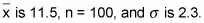2. Take 11.5 – 11 = +0.5.

3. Take 2.3 divided by the square root of 100 (which is 10) to get 0.23 for the standard error.

4. Divide +0.5 by 0.23 to get 2.17. That’s your test statistic, which means your sample mean is 2.17 standard errors above the claimed population mean.

The big idea of a hypothesis test is to challenge the claim that’s being made about the population (in this case, the population mean); that claim is shown in the null hypothesis, H0. If you have enough evidence from your sample against the claim, H0 is rejected.

To decide whether you have enough evidence to reject H0, calculate the p-value by looking up your test statistic (in this case 2.17) on the standard normal (Z-) distribution — see the above Z-table — and take 1 minus the probability shown. (You subtract from 1 because your Ha is a greater-than hypothesis and the table shows less-than probabilities.)

For this example you look up the test statistic (2.17) on the Z-table and find the (less-than) probability is 0.9850, so the p-value is 1 – 0.9850 = 0.015. It’s quite a bit less than your (typical) significance level 0.05, which means your sample results are quite statistically significant. So reject the claim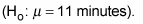Your results support the alternative hypothesis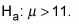According to your data, the child psychologist’s claim of 11 minutes per day is too low; the actual average is greater than that.

The temptation is to say, “Well, I knew the claim of 11 minutes per day was too low because the sample mean of 11.5 minutes was clearly larger. Why do I even need a hypothesis test?” All that number tells you is something about those 100 mothers sampled. You also need to factor in variation using the standard error and the normal distribution to be able to say something about the entire population of working mothers.